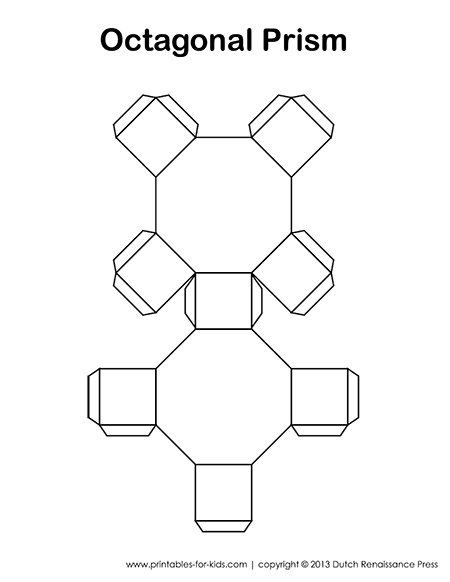# Octagonal Prisms: Paper Models, Surface Area, Volume Formulas and Nets

Everything you ever wanted to know about octagonal prisms. Whether you’re a student learning about polyhedrons for the first time, or a parent who needs a recap, by the time you get to the end of this webpage, you’ll be an expert.## Octagonal Prism Definition

An octagonal prism is a 3D object with two regular octagon caps and rectangular or square sides.

## Octagonal Prism Paper Model and Net

Below is a free printable octagonal prism net. Please open the pdf file by clicking on the image below. Then you will be able to download the file in your browser. (If you are unable to see the PDF, you may need an updated version of Adobe Acrobat Reader.)## Construction Tutorial1. Print the template on some sturdy construction paper.2. Cut out the prism net along its perimeter. Fold along the lines of the flaps and shapes that make up the net.3. Fold the pieces of the prism so that the octagon caps are facing. Tape the flaps under the faces with scotch tape. Once all the flaps are taped, your octagonal prism paper model is complete.

[clearBoth]

## Octagonal Prism Surface Area FormulasOctagonal Prism Surface Area Formula:
8 R+ 2B
Where:
R = the Area of a Rectangle Face
B = the Area of an Octagon Face

The surface area of a prism is equal to the sum of the areas of its faces. An octagonal prism is made up of 8 rectangle faces and 2 octagon faces.

If you happen to know R and B, then you’re all done. But what if you only know the length of a few sides of the octagonal prism? No problem. Let’s take a look.Octagonal Prism Surface Area Formula:
Where:
a = the length of an octagon edge
l = the length of the octagonal prism

d = the width of an octagon face

Notice that the first and second formulas are actually the same, since:

R =al

Instead of 8R +2B, we get: 8(al) + 2(2ad) which is equal to the second formula: 8al+ 4ad

## Octagonal Prism Volume FormulasOctagonal Prism Volume Formula:
Volume =Bl
Where:
B = the Area of an Octagon Face
l = the length of the octagonal prism

Octagonal Prism Volume Formula:
Where:
a = the length of an octagon edge
l = the length of the octagonal prism
d = the width of an octagon face

Notice that the first and second formulas are actually the same, since:

## Vertices, Faces, and Edges

An octagonal prism has 16 vertices, 10 faces, and 24 edges.

### 1 thought on “Octagonal Prisms: Paper Models, Surface Area, Volume Formulas and Nets”

1.Rune Sirevåg

I find it useful